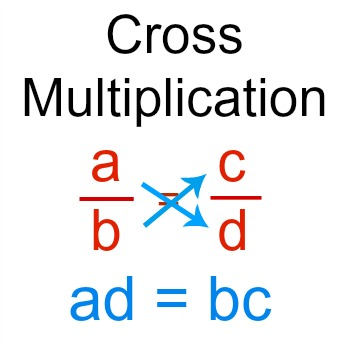# How do you solve  ( x+12 )/( x-4 ) = ( x-3 )/( x-7 ) ?

Apr 7, 2018

$x = 8$

#### Explanation:

First, we'll cross multiply: if $\frac{a}{b} = \frac{c}{d}$ then $a \cdot d = b \cdot c$

$\left(x + 12\right) \left(x - 7\right) = \left(x - 4\right) \left(x - 3\right)$

Expand

${x}^{2} + 5 x - 84 = {x}^{2} - 7 x + 12$

Add 84 to both sides and simplify

${x}^{2} + 5 x \cancel{- 84 + 84} = {x}^{2} - 7 x + 12 + 84$
${x}^{2} + 5 x = {x}^{2} - 7 x + 96$

Subtract ${x}^{2} - 7 x$ from both sides and simplify

${x}^{2} + 5 x - \left({x}^{2} - 7 x\right) = {x}^{2} - 7 x + 96 - \left({x}^{2} - 7 x\right)$
$12 x = 96$

Divide both sides by 12 and simplify

$\frac{\cancel{12} x}{\cancel{12}} = \frac{96}{12}$
$x = 8$

~ Alex

Apr 7, 2018

$x = 8$

#### Explanation:

To solve this, we use cross multiplication:Based on this, in this case you would do:
$\left(x + 12\right) \left(x - 7\right) = \left(x - 3\right) \left(x - 4\right)$

And now we just expand and simplify:
${x}^{2} + 12 x - 7 x - 84 = {x}^{2} - 3 x - 4 x + 12$

Combine like terms:
${x}^{2} + 5 x - 84 = {x}^{2} - 7 x + 12$

Subtract ${x}^{2}$ on both sides of the equation:
${x}^{2} \textcolor{red}{- {x}^{2}} + 5 x - 84 = {x}^{2} \textcolor{red}{- {x}^{2}} - 7 x + 12$

$5 x - 84 = - 7 x + 12$

Add $7 x$ to both sides of the equation:
$5 x \textcolor{red}{+ 7 x} - 84 = - 7 x \textcolor{red}{+ 7 x} + 12$

$12 x - 84 = 12$

Add $84$ to both sides of the equation:
$12 x - 84 \textcolor{red}{+ 84} = 12 \textcolor{red}{+ 84}$

$12 x = 96$

Divide both sides by $12$:
$x = 8$

Hope this helps!